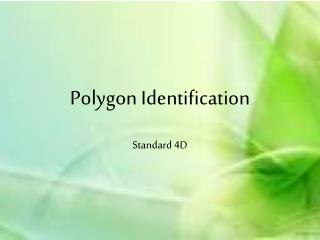DownloadDownload PresentationPolygon Identification

# Polygon Identification

Télécharger la présentation## Polygon Identification

- - - - - - - - - - - - - - - - - - - - - - - - - - - E N D - - - - - - - - - - - - - - - - - - - - - - - - - - -
##### Presentation Transcript

1. Polygon Identification Standard 4D

2. A regular polygon is a polygon whose sides are all the same length, and whose angles are all the same measure. The sum of the angles of a polygon with n sides, where n is 3 or more, is 180° × (n - 2)

3. Triangle - A three-sided polygon. The sum of the angles of a triangle is 180° • Equilateral - All sides are the same length. • Equiangular - All angles have the same measure. • Regular polygons are both equilateral and equiangular.

4. Quadrilateral - A four-sided polygon. The sum of the angles of a quadrilateral is 360°

5. Pentagon - A five-sided polygon. The sum of the angles of a pentagon is 540°

6. Hexagon - A six-sided polygon. The sum of the angles of a hexagon is 720°

7. Heptagon - A seven-sided polygon. The sum of the angles of a heptagon is 900°

8. Octagon - An eight-sided polygon. The sum of the angles of an octagon is 1080°

9. Nonagon - A nine-sided polygon. The sum of the angles of a nonagon is 1260°

10. Decagon - A ten-sided polygon. The sum of the angles of a decagon is 1440°